# GSEB Solutions Class 12 Maths Chapter 13 Probability Ex 13.4

Gujarat Board GSEB Textbook Solutions Class 12 Maths Chapter 13 Probability Ex 13.4 Textbook Questions and Answers.

## Gujarat Board Textbook Solutions Class 12 Maths Chapter 13 Probability Ex 13.4

Question 1.
State which of the following are not probability distribution of a random variable. Give reasons for your answers.Solution:
(i) Sum of probabilities = 0.4 + 0.4 + 0.2 = 1.
∴ This distribution is a probability deistribution.

(ii) One of the probabilities – 0.1 is negative.
∴ It is not a probability distribution.

(iii) Sum of probabilities is 0.6 + 0.1 + 0.1 = 0.9 ≠ 1.
∴ The given distribution is not a probability distribution.

(iv) Sum of probabilities = 0.3 + 0.2 + 0.4 + 0.1 + 0.5 = 1.05 > 1
∴ It is not a probability distribution.Question 2.
An urn contains 5 red and 2 black balls. Two balls are randomly drawn. Let X represents the number of black balls. What are the possible values of X, if X is a random variable?
Is X a random variable?
Solution:
These two balls may be selected as RR, RB, BR, BB, where R represents red and B represents black ball.
Variable X has the value 0,1,2, i.e., there may be no black balls, may be one black ball, or both the balls are black.
Yes, X is a random variable.

Question 3.
Let X represents the difference between the number of heads and the number of tails obtained, when a coin is tossed 6 times.
What are the possible values of X?
Solution:
When a coin is tossed 6 times, we obtain the number of heads and tails as follows:
Corresponding values of X are shown here.Question 4.
Find the probability distribution of:
(i) number of heads in two tosses of a coin.
(ii) number of tails in the simultaneous tosses of three coins.
(iii) number of heads in four tosses of a coin.
Solution:
(i) When two tosses of a coin are there:
Sample space = {TT, TH, HT, HH}
Zero success ⇒ No head ⇒ Two tails (TT)
∴ P(0) = $$\frac{1}{2}$$ × $$\frac{1}{2}$$ = $$\frac{1}{4}$$.
One success ⇒ 1 head or 1 tail (TH, HT)
P(1) = $$\frac{1}{2}$$ × $$\frac{1}{2}$$ + $$\frac{1}{2}$$ × $$\frac{1}{2}$$
= $$\frac{1}{4}$$ + $$\frac{1}{4}$$
= $$\frac{1}{2}$$.
Two success ⇒ Both heads (HH)
P(2) = $$\frac{1}{2}$$ × $$\frac{1}{2}$$ = $$\frac{1}{4}$$.
∴ Probability Distribution(ii) When three coins are tossed simultaneously:
Sample space = {TTT, TTH, THT, HTT, THH, HTH, HHT, HHH}
Zero success = No tail = All head {HHH}
∴ P(0) = $$\frac{1}{2}$$ × $$\frac{1}{2}$$ × $$\frac{1}{2}$$ = $$\frac{1}{8}$$.
1 success = 1 tail and 2 heads
= {THH, HTH, HHT}
∴ P(1) = 3 × $$\frac{1}{2}$$ × $$\frac{1}{2}$$ × $$\frac{1}{2}$$ = $$\frac{3}{8}$$.
1 success = 1 tail and 2 heads
= {THH, HTH, HHT}
2 success = 2 tail and 1 head
= {TTH, THT, HTT}
P(2) = 3 × $$\frac{1}{2}$$ × $$\frac{1}{2}$$ × $$\frac{1}{2}$$ = $$\frac{3}{8}$$.
3 success = All the tails = {TTT}
P(3) = $$\frac{1}{2}$$ × $$\frac{1}{2}$$ × $$\frac{1}{2}$$ = $$\frac{1}{8}$$.
∴ Probability distribution is:(iii) When a coin is tossed 4 times:
Zero success = No head = All are tails {TTTT}
= $$\frac{1}{2}$$ × $$\frac{1}{2}$$ × $$\frac{1}{2}$$ × $$\frac{1}{2}$$ = $$\frac{1}{16}$$.
1 success = 1 head 3 tails
= {HTTT, THTT, TTHT, TTTH}
P(1) = 4C2 × $$\frac{1}{2}$$ × $$\frac{1}{2}$$ × $$\frac{1}{2}$$ × $$\frac{1}{2}$$ = $$\frac{4}{16}$$ = $$\frac{1}{4}$$.
2 success = 2 heads and 2 tails
= {HHTT, HTHT, HTTH, THHT, THTH, TTHH}
∴ P(2) = 4C2 × $$\frac{1}{2}$$ × $$\frac{1}{2}$$ × $$\frac{1}{2}$$ × $$\frac{1}{2}$$ = $$\frac{6}{16}$$ = $$\frac{3}{8}$$.
3 successses = 3 heads and 1 tail
= {HHHT, HHTH, HTHH, THHH}
∴ P(3) = 4C3 × $$\frac{1}{2}$$ × $$\frac{1}{2}$$ × $$\frac{1}{2}$$ × $$\frac{1}{2}$$ = $$\frac{4}{16}$$ = $$\frac{1}{4}$$.
4 successes = All the heads = {HHHH}
P(4) = $$\frac{1}{2}$$ × $$\frac{1}{2}$$ × $$\frac{1}{2}$$ × $$\frac{1}{2}$$ = $$\frac{1}{16}$$.
Thus, the probability distribution is: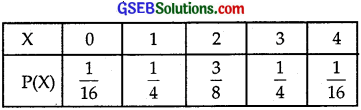Question 5.
Find the probability distribution of the number of successses in two tosses of a dice, where a success is defined as
(i) number greater than 4.
(ii) six appeared on at least one dice.
Solution:
(i) When a number on each dice is 1, 2, 3, 4.
Such cases are: {(1, 1), (1, 2), ……………, (2, 1), (2, 2), ……………., (4, 4))} = 16 cases
Total number of cases in sample space
= 6 × 6 = 36.
∴ P(0) = $$\frac{16}{36}$$ = $$\frac{4}{9}$$.
1 success = 5 or 6 on one dice and 1, 2, 3, 4 on the other dice = 16
∴ P(1) = $$\frac{16}{36}$$ = $$\frac{4}{9}$$.
Two successes = 5 or 6 on both dice
= (5, 6), (6, 5), (5, 5), (6, 6)
∴ P(2) = $$\frac{4}{36}$$ = $$\frac{1}{9}$$.
∴ Probability distribution is as follows: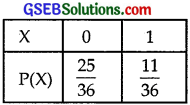(ii) Zero success = 6 does not appear on any dice.
= 1, 2, 3, 4, 5 appear on both sides
∴ Number of such cases = 25
P(0) = $$\frac{25}{36}$$.
1 success ⇒ 6 appears on one dice and 1, 2, 3, 4, 5 appears on both dice
∴ Number of such cases = 25
P(0) = $$\frac{25}{36}$$.
1 success ⇒ 6 appears on one dice and 1, 2, 3, 4, 5 appears on the other dice.
Number of cases = 10.
2 successes ⇒ 6 appears on both dice {6, 6}
Number of such cases = 1.
Number of cases, when there is at least one ‘six’ appears
= 10 + 1 = 11.
∴ Probability of getting at least 1 six = $$\frac{11}{36}$$.
∴ Probability distribution is:Question 6.
From a lot of 30 bulbs, which include 6 defectives, a sample of 4 bulbs is drawn at random with replacement.
Find the probability distribution of number of defective bulbs.
Solution:
There are 30 bulbs, which include 6 defective bulbs.
Probability of getting a defective bulb = $$\frac{6}{30}$$ = $$\frac{1}{5}$$.
Probability of getting a good bulb = 1 – $$\frac{1}{5}$$ = $$\frac{4}{5}$$.
Let X denotes variable of defective bulbs in a sample of 4 bulbs.∴ Probability distribution of defective bulbs is:Question 7.
A com is biased so that the head is 3 times as likely to occur as tail. If the coin is tossed twice, find the probability distribution of tails.
Solution:
When the coin is tossed, the head occurs 3 times the tail occurs.
Let the tail occurs x times.
So, total number of cases = x + 3x = 4x.
∴ Probability of getting a head = $$\frac{3x}{4x}$$ = $$\frac{3}{4}$$.
∴ Probability of getting a tail = $$\frac{x}{4x}$$ = $$\frac{1}{4}$$.
When no tails occurs, the probability of getting {HH}
= $$\frac{3}{4}$$ × $$\frac{1}{4}$$ + $$\frac{1}{4}$$ × $$\frac{3}{4}$$ = $$\frac{3}{16}$$ × 2 = $$\frac{3}{8}$$.
The probability of getting both tails
P(2) = $$\frac{1}{4}$$ × $$\frac{1}{4}$$ = $$\frac{1}{16}$$.
∴ Probability distribution of occuring tails: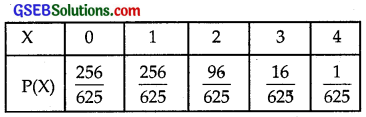Question 8.
A random variable X has the following probability distribution: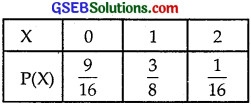Determine:
(i) k’
(ii) P(X< 3) (iii) P(X > 0)
(iv) P(0 < X < 3)
Solution:
(i) Sum of probabilities = 1
i.e; o + k + 2k + 2k + 3k + k2 + 2k2 + 7k2 + k = 1
or 10k2 + 9k = 1 or 10k2 + 9k – 1 = 0
⇒ (k + 1)(10k – 1) = 0
∴ k = – 1 or k = $$\frac{1}{10}$$
k ≠ – 1 ∴ k = $$\frac{1}{10}$$
∴ The probability distribution is:Question 9.
The random variable X has a probability distribution P(X) of the following form, where k is some number: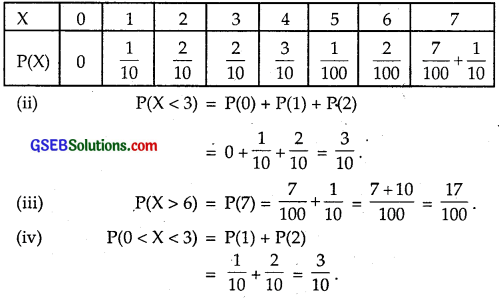(a) Determine the value of k.
(b) Find P(X < 2), P(X ≤ 2), P(X ≥ 2).
Solution:
(a) Sum of probabilities = 1
∴ k + 2k + 3k = 1 or 6k = 1
⇒ k = $$\frac{1}{6}$$.
The probability distribution is as given below: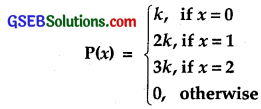Question 10.
Find the mean number of heads in three tosses of a fair coin.
Solution:
Let H denotes the success of getting a head.
Sample space {TTT, THH, THT, HTT, THH, HTH, HHT, HHH}
Zero success = {TTT}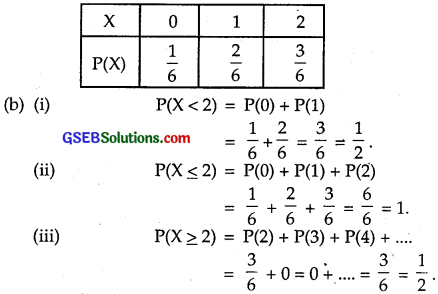Question 11.
Two dice are thrown simultaneously. If X denotes the number of sixes, find the expectation of X.
Solution:
Let X denotes the number sixes.
Probability of getting one six on one dice = $$\frac{1}{6}$$.
Probability of getting 1, 2, 3, 4 or 5 on a dice = $$\frac{5}{6}$$.
0 success ⇒ Six does not appear on any dice.
∴ Probability of getting 1, 2, 3, 4, 5 on both dice
= $$\frac{5}{6}$$ × $$\frac{5}{6}$$ = $$\frac{25}{36}$$.
i.e., P(0) = $$\frac{25}{36}$$.
One six on one of the dice will occur as (Six, Non-six), (Non- six, Six).
∴ Probability of getting a success
P(1) = $$\frac{1}{6}$$ × $$\frac{5}{6}$$ + $$\frac{5}{6}$$ × $$\frac{1}{6}$$ = $$\frac{10}{36}$$.
Probability of getting sixes on both dice
P(2) = $$\frac{1}{6}$$ × $$\frac{1}{6}$$ = $$\frac{1}{36}$$.
∴ Probability distribution is:Question 12.
Two numbers are selected at random (without replacement) from the first six positive integers.
Let X denotes the larger of the two numbers obtained. Find E(X).
Solution:
There are six numbers 1, 2, 3, 4, 5, 6.
One of them is selected in 6 ways.
When one of the numbers has been selected, 5 numbers are left.
One number out of 5 may be selected in 5 ways.
∴ No. of ways of selecting two numbers without replacement out of 6 positive integers = 6 × 5 = 30.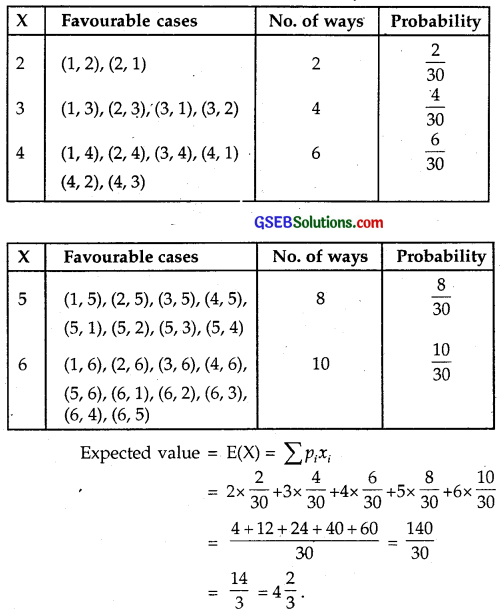Question 13.
Let X denotes the sum of the numbers obtained, when two fair dice are rolled. Find the variance and standard deviation of X.
Solution:
When two dice are rolled, the number of exhaustive cases = 6 × 6 = 36.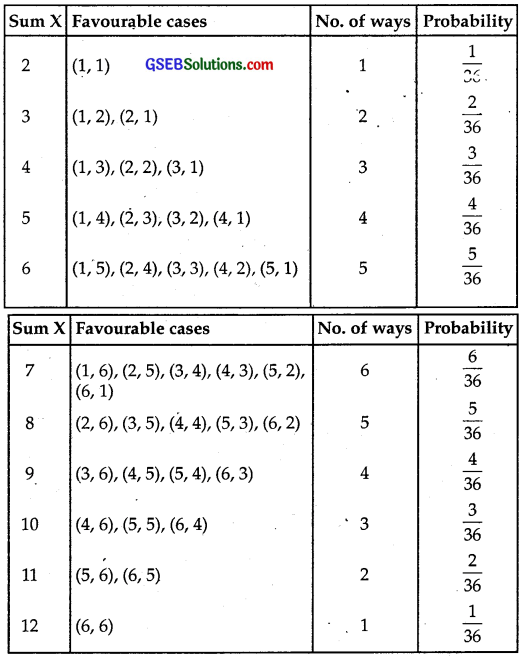∴ Probability distribution:Question 14.
A class has 15 students whose ages are 14, 17, 15, 14, 21, 17, 19, 20, 16, 18, 20, 17, 16, 19 and 20 years.
One student is selected in such a manner that each has the same chance of being chosen and the age X of the selected student is recorded. What is the probability distribution of the random variable X?
Find the mean, variance and standard deviation of X.
Solution:
There are 15 students in a class. Each has the same chance of being choosen.
The probability of each student to be selected = $$\frac{1}{15}$$.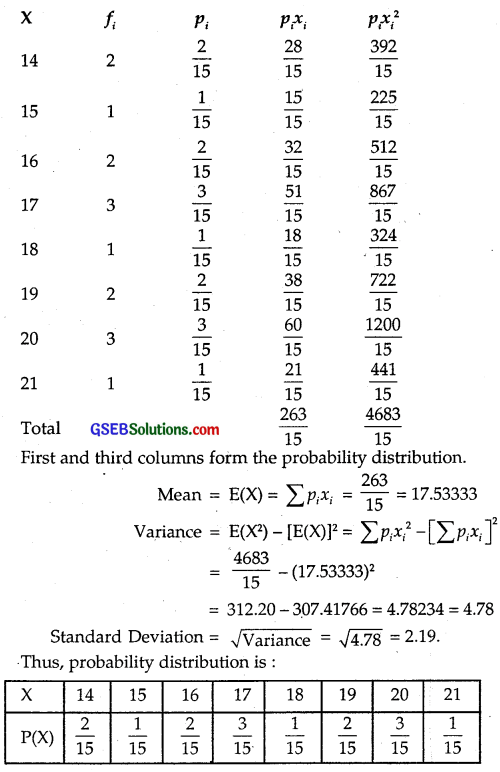Mean = 17.53, Variance = 4.78, S.D. (X) = 2.19.

Question 15.
In a meeting, 70% of the members favojir and 30% oppose a certain proposal. A member is selected at random and we take X = 0, if he opposed and X = 1, if he is in favour.
Find E(X) and Var(X).
Solution:
Here, the variable values are 1 and 0 and the probability of occurrence is 70% = 0.7 and 30% = 0.3.
Probability distribution is: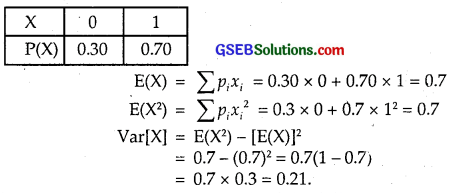Choose the correct answer in each of the following:

Question 16.
The means of the numbers obtained on throwing a dice having written 1 on three faces, 2 on two faces and 5 on one face is
(A) 1
(B) 2
(C) 3
(D) $$\frac{8}{3}$$
Solution:
The variables are 1, 2 and 5.
1 is written on 3 faces.
∴ Probability of getting 1 = $$\frac{3}{6}$$ = $$\frac{1}{2}$$.
2 is written on two faces.
∴ Probablility of getting 2 = $$\frac{2}{6}$$ = $$\frac{1}{3}$$.
5 is written on one face.
∴ Probability of getting 5 = $$\frac{1}{6}$$.
Probability distribution is:∴ Part (B) is the correct answer.Question 17.
Suppose that two cards are drawn at random from a deck of cards. Let X be the number of aces obtained. Then, value of E(X) is
(A) $$\frac{37}{221}$$
(B) $$\frac{27}{221}$$
(C) $$\frac{1}{13}$$
(D) $$\frac{2}{13}$$
Solution:
(i) When two cards are drawn, let no ace occurs.
Two non ace cards may be drawn in 48C2 ways
= $$\frac{48×47}{2}$$ = 24 × 47 = 1128.
Out of 52 cards, any two cards may be drawn in 52C2
= $$\frac{52×51}{2}$$ = 26 × 51 = 1326 ways
∴ Probability of getting no ace = $$\frac{1128}{1326}$$.

(ii) One ace and one non-ace cards may be drawn in
4C1 × 48C1 = 4 × 48 = 192 ways.
∴ Probability of getting one ace and one non-ace card
= $$\frac{1128}{1326}$$.

(iii) The number of ways of drawing two aces = 4C2 = 6
∴ Probability of getting 2 aces = $$\frac{6}{1326}$$.
∴ Probability distribution is: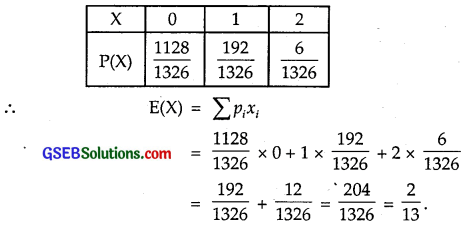∴ Part (D) is the correct answer.